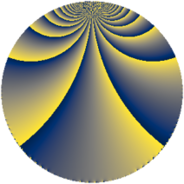# Properties

 Label 324.3.dLevel $324$ Weight $3$ Character orbit 324.d Rep. character $\chi_{324}(163,\cdot)$ Character field $\Q$ Dimension $44$ Newform subspaces $9$ Sturm bound $162$ Trace bound $2$

# Related objects

## Defining parameters

 Level: $$N$$ $$=$$ $$324 = 2^{2} \cdot 3^{4}$$ Weight: $$k$$ $$=$$ $$3$$ Character orbit: $$[\chi]$$ $$=$$ 324.d (of order $$2$$ and degree $$1$$) Character conductor: $$\operatorname{cond}(\chi)$$ $$=$$ $$4$$ Character field: $$\Q$$ Newform subspaces: $$9$$ Sturm bound: $$162$$ Trace bound: $$2$$ Distinguishing $$T_p$$: $$5$$

## Dimensions

The following table gives the dimensions of various subspaces of $$M_{3}(324, [\chi])$$.

Total New Old
Modular forms 120 52 68
Cusp forms 96 44 52
Eisenstein series 24 8 16

## Trace form

 $$44 q + 2 q^{4} + O(q^{10})$$ $$44 q + 2 q^{4} + 4 q^{10} + 4 q^{13} - 10 q^{16} + 78 q^{22} + 144 q^{25} + 48 q^{28} - 2 q^{34} + 16 q^{37} + 112 q^{40} - 60 q^{46} - 136 q^{49} - 44 q^{52} + 52 q^{58} + 124 q^{61} - 262 q^{64} - 168 q^{70} + 16 q^{73} - 54 q^{76} - 50 q^{82} - 64 q^{85} - 306 q^{88} - 336 q^{94} + 124 q^{97} + O(q^{100})$$

## Decomposition of $$S_{3}^{\mathrm{new}}(324, [\chi])$$ into newform subspaces

Label Dim. $$A$$ Field CM Traces $q$-expansion
$$a_2$$ $$a_3$$ $$a_5$$ $$a_7$$
324.3.d.a $$2$$ $$8.828$$ $$\Q(\sqrt{3})$$ $$\Q(\sqrt{-1})$$ $$-4$$ $$0$$ $$-8$$ $$0$$ $$q-2q^{2}+4q^{4}+(-4+\beta )q^{5}-8q^{8}+\cdots$$
324.3.d.b $$2$$ $$8.828$$ $$\Q(\sqrt{-3})$$ None $$-2$$ $$0$$ $$8$$ $$0$$ $$q+(-1-\zeta_{6})q^{2}+(-2+2\zeta_{6})q^{4}+4q^{5}+\cdots$$
324.3.d.c $$2$$ $$8.828$$ $$\Q(\sqrt{-3})$$ None $$2$$ $$0$$ $$-8$$ $$0$$ $$q+(1+\zeta_{6})q^{2}+(-2+2\zeta_{6})q^{4}-4q^{5}+\cdots$$
324.3.d.d $$2$$ $$8.828$$ $$\Q(\sqrt{3})$$ $$\Q(\sqrt{-1})$$ $$4$$ $$0$$ $$8$$ $$0$$ $$q+2q^{2}+4q^{4}+(4+\beta )q^{5}+8q^{8}+(8+\cdots)q^{10}+\cdots$$
324.3.d.e $$6$$ $$8.828$$ 6.0.3636603.2 None $$-1$$ $$0$$ $$-2$$ $$0$$ $$q-\beta _{3}q^{2}-\beta _{1}q^{4}+(-\beta _{1}+\beta _{2}-\beta _{3}+\cdots)q^{5}+\cdots$$
324.3.d.f $$6$$ $$8.828$$ 6.0.3636603.2 None $$1$$ $$0$$ $$2$$ $$0$$ $$q-\beta _{1}q^{2}-\beta _{2}q^{4}+(-\beta _{1}+\beta _{2}-\beta _{3}+\cdots)q^{5}+\cdots$$
324.3.d.g $$8$$ $$8.828$$ 8.0.$$\cdots$$.1 None $$-3$$ $$0$$ $$6$$ $$0$$ $$q-\beta _{1}q^{2}+(\beta _{1}+\beta _{2}+\beta _{5}-\beta _{6})q^{4}+\cdots$$
324.3.d.h $$8$$ $$8.828$$ 8.0.$$\cdots$$.4 None $$0$$ $$0$$ $$0$$ $$0$$ $$q+\beta _{3}q^{2}+(-1-\beta _{6})q^{4}+(-2\beta _{1}+\beta _{2}+\cdots)q^{5}+\cdots$$
324.3.d.i $$8$$ $$8.828$$ 8.0.$$\cdots$$.1 None $$3$$ $$0$$ $$-6$$ $$0$$ $$q+\beta _{6}q^{2}+(-\beta _{1}+\beta _{2}+\beta _{4}+\beta _{6})q^{4}+\cdots$$

## Decomposition of $$S_{3}^{\mathrm{old}}(324, [\chi])$$ into lower level spaces

$$S_{3}^{\mathrm{old}}(324, [\chi]) \cong$$ $$S_{3}^{\mathrm{new}}(12, [\chi])$$$$^{\oplus 4}$$$$\oplus$$$$S_{3}^{\mathrm{new}}(36, [\chi])$$$$^{\oplus 3}$$$$\oplus$$$$S_{3}^{\mathrm{new}}(108, [\chi])$$$$^{\oplus 2}$$